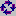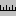Key Words Plot Display
 KeyWords > plot display   The plot will give you useful visual insights into how often and where the different key words crop up in the text. The plot is initially sorted to show which crop up more at the beginning (e.g. in the introduction) and then those from further in the text.   The following screenshot shows KWs of the Bible, revealing where each term occurs. The name Jehoshaphat, for example, occurs mainly about one third of the way through the text.re-sorting You can re-sort the listing using. Re-sorting rotates through the following types: first mention of each key word in the text dispersion within the text the original plot order (which is based on key-ness) alphabetical order total number of links with other key-words   links This shows the total number of links between the key-word and other key-words in the same text, within the current collocation span (default = 5,5). That is, how many times was each key-word found within 5 words of left or right of any of the other key-words in your plot.   hits This column is here to remind you of how many occurrences there were of each key-word. When you have obtained a plot, you can then see the way certain words relate to others. To do this, look at the Links window in the tabs at the bottom, showing which other key words are most linked to the word you clicked on. That is, which other words occur most often within the collocation horizons you've set. The Links window should help you gain insights into the lexical relations here. Each plot window is dependent on the key words listing from which it was derived. If you close that down, it will disappear. You can Print it. There's no Save option because the plot comes from a key words listing which you should Save, or Save As. There's no save as text option because the plot has graphics, which cannot adequately be represented as text symbols, but you can Copy to the clipboard (Ctrl-Ins) and then paste it into a word processor as a graphic. Alternatively, use the Output | Data as Text File option, which saves your plot data (each word is followed by the total number of words in the file, then the word number position of each occurrence). The ruler in the menu () allows you to see the plot divided into 8 equal segments if based on one text, or the text-file divisions if there is more than one.   See also: Key words plot, plot dispersion value